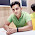# Binary to Decimal C Program

The formula of Binary to Decimal is:
Decimal Number = (nth digit of binary number) * 2(n-1) + ((n-1)th digit of binary number) * 2(n-2) + …… + (1st digit of binary number) * 2(0)

Suppose 1101 is the binary number. Then the decimal number will be:
Decimal Number = (1 * 23 + 1 * 22 + 0 * 21 + 1* 20) = (8 + 4 + 0 + 1) = 13

Here is the C program to convert Binary Number into Decimal Number:

```#include <stdio.h>
#include <math.h>

int main()
{
int binary, decimal = 0, i, j=0;
printf("Enter a binary number: ");
scanf("%d",&binary);
int temp;
temp = binary;
if(temp>0){
i = temp % 10;
if(i==0 || i==1){
while(temp!=0){
i = temp % 10;
decimal = decimal + i * pow(2, j);
temp = temp / 10;
j++;
}
}
}
if(decimal==0 || binary<0)
printf("The number is not a binary number !");
else printf("The equivalent decimal number of %d is : %d.",binary,decimal);
return 0;
}```

NB: Here pow(x,y) is a function that comes with header file math.h. It returns x to the power y.
Hope you have enjoyed this program. Please share it with your friends if you have liked this.
Share:

#### 1 comments :

1.Spam comments will be deleted. :)